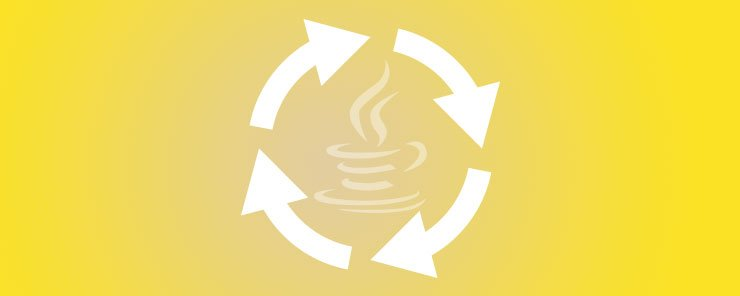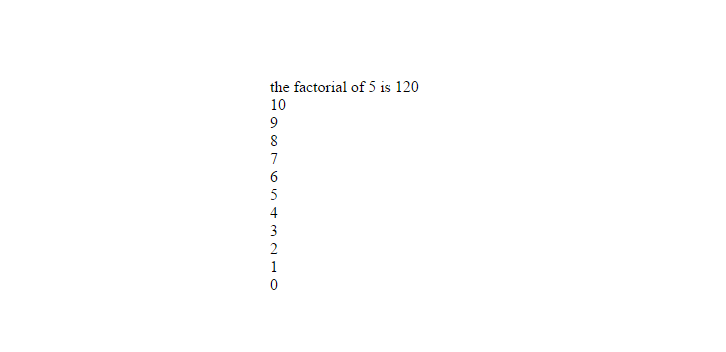Web Programming TutorialsLearn about Recursive Functions in JavaScript

# Learn about Recursive Functions in JavaScriptA recursive function is basically a function that calls itself. A recursive function always includes an if statement. There must be at least 2 options: a scenario where the function calls itself (the recursive call) and a default scenario where the function does not call itself and the recursion ends.
The default scenario is the simplest case where no looping follows.
In the recursive scenario, if the recursive call returns a value, we usually store it in a variable.
When looking at a recursive function, a step in understanding its purpose is asking ourselves – how does solving the smaller problem (the recursive functionality) help in solving the bigger problem(the function’s ultimate purpose).

Lets construct a recursive function as an example. Our function will take a positive integer n as a parameter and calculate the number of possible ways to organize n different objects in a sequence. In mathematical terms, this number is called the factorial , and it’s denoted by n!
The general forumla for calculating a factorial for a number n is 1*2*(n-k)*….*(n-3)*(n-2)*(n-1)*n
Note: n!=1 both for n=1 and for n=0.

```function Recur(n){

if (n<=1){
return 1;
}
else{
return n*Recur(n-1);
}

}
```

Explanation:
The function first checks for the default scenario – is n smaller or equal to 1. If so, it returns 1 which is the factorial of 1 and 0; Otherwise, it runs the recursive call – it calls itself on n-1.

To better understand this process, lets look at a stage by stage break-down of the loop for the case of n=5 or Recur(5).

 round number 1 2 3 4 5 if statement FALSE FALSE FALSE FALSE TRUE returned value 5* Recur(4) 4*Recur(3) 3*Recur(2) 2*Recur(1) 1

Recur(5) runs recursively until n<=1 is true, in which case it returns 1 to stage 4, which doubles it and returns 2 to stage 3, which triples it and returns 6 to stage 2, which quadruples it and returns 24 to stage 1, which quintuples it and returns 120.

Lets try out our function by testing the output for n=5:

`alert( 'the factorial of 5 is ' + Recur(5));`Lets look at another example of a simple recursive function. We can write a recursive function to do a countown from a given number n until 0, like so:

```function Recur2(n){
document.write(n + '<br/>');
if (n<=0){
return;
}
else{
return Recur2(n-1);
}

}
```

Explanation: Recur2 takes a number n as a parameter. It starts by printing out that number (unconditionally), and only then comes the if statement, followed by the default scenario – if n is zero or negative, the instruction to the function is to stop (do nothing).

If the default scenario is false (n is bigger than 0), we move on to the recursive call and the function calls itself on n-1.

Lets call our function to see if it works as expected:

`document.write(Recur2(10));`### To summarize,

Recursion is basically a situation where a function keeps calling itself until a default condition is met, afterwhich it doesn’t call itself anymore(this condition must exist, otherwise it will cause an infinite loop). Every recursive function can be written as a loop instead, but not every loop can be written as a recursive function. There are a few things you can only do with recursive functions.

### Top 10 cyber security threats that arose last year

21,501Fans
4,220Followers
103,000Subscribers

### Top 10 cyber security threats that arose last year

- Advertisement -